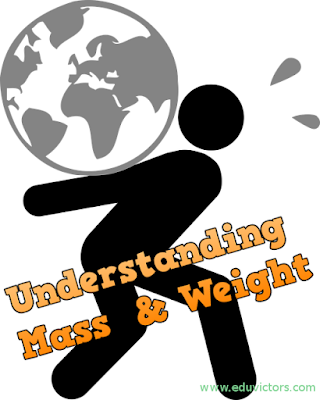## Pages

Showing posts with label class11-physics. Show all posts
Showing posts with label class11-physics. Show all posts

# Class 11 - Physics - Mechanical Properties of Solids and Fluids - Important Points to Remember

1. Elasticity:

It is the property of a material by which it tries to regain its original configuration after the removal of the deforming force applied to it. Example of perfectly elastic solid, quartz, phosphor, bronze.

2. Plasticity:

It is the property of a body by virtue of which it does not regain its original shape and size even after the removal deforming force, is called plasticity.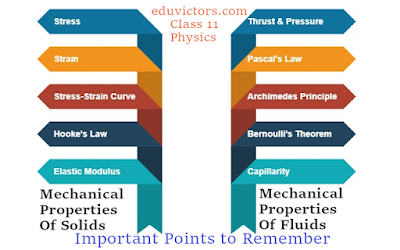# PHYSICS MCQs FOR NEET - IIT JEE EXAMINATION (SET-6)

Topics Covered: Work, Power and Energy

Q1: A bullet is fired from a rifle, If the rifle recoils freely, then the kinetic energy of the rifle will be:

(a) same as that of bullet

(b) more than that of bullet

(c) less than that of bullet

(d) none of these

Q2: A body of mass 2 kg, initially at rest, is acted upon simultaneously by two forces, one of 4N and the other of 3N, acting at right angles to each other. The work done by the body after the 20s is

(a) 500 J

(b) 1250 J

(c) 2500 J

(d) 5000 J# CBSE Class 11 - Physics Annual Question Paper Set-3(2020-21)

For a large number of students, the higher secondary stage will be a preparation for the university, where a fairly high degree of proficiency in Physics may be required. But for another large group, the higher secondary stage may be a preparation for entry into the professional domain like engineering and research. It is expected that the syllabus will help to develop an interest in the learners to study Physics as a discipline and inculcate in learners the abilities, useful concepts of Physics in real-life situations for making learning of Physics relevant, meaningful and interesting.

Here follows the class 11  Physics  - Annual Sample question paper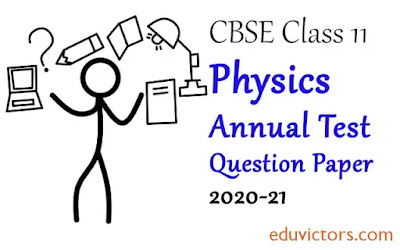# CBSE Class 11 - Physics Annual Question Paper Set-2(2020-21)

For a large number of students, the higher secondary stage will be a preparation for the university, where a fairly high degree of proficiency in Physics may be required. But for another large group, the higher secondary stage may be a preparation for entry into the professional domain like engineering and research. It is expected that the syllabus will help to develop an interest in the learners to study Physics as a discipline and inculcate in learners the abilities, useful concepts of Physics in real-life situations for making learning of Physics relevant, meaningful and interesting.

Here follows the class 11  Physics  - Annual Sample question paper# CBSE Class 11 - Physics Annual Question Paper(2020-21)

For a large number of students, the higher secondary stage will be a preparation for the university, where a fairly high degree of proficiency in Physics may be required. But for another large group, the higher secondary stage may be a preparation for entry into the professional domain like engineering and research. It is expected that the syllabus will help to develop an interest in the learners to study Physics as a discipline and inculcate in learners the abilities, useful concepts of Physics in real-life situations for making learning of Physics relevant, meaningful and interesting.

Here follows the class 11  Physics  - Annual Sample question paper# Physicists and Their Major Contributions

For Class 11/Class 12 Physics - NEET - JEE

Physics deals with the study of the basic laws of nature and their manifestation in different phenomena. The basic laws of physics are universal and are applied in widely different contexts and conditions. Physics is a basic discipline in the category of natural sciences which also includes other disciplines like Chemistry and Biology. The word physics comes from a Greek word meaning nature.

Here is a partial list of Physicists and their contributions in various fields of physics.# JEE NEET PHYSICS MCQs (Set-5)

Topics Covered: Laws of Motion, Circular Motion

Q1: In a tug-of-war match, one team is pulling with a force of 500 N. If they are exactly balanced by the other team, then the tension in the rope is?

(a) 0
(b) 250 N
(c) 500 N
(d) 1000 N

Q2: A particle of mass 0.3 kg is subjected to a force F = kx with k= 15 N/m. What will be its initial acceleration if it is released from a point 20 cm away from the origin.

(a) 3 m/s²
(b) 15 m/s²
(c) 5m/s²
(d) 10 m/s²# JEE NEET PHYSICS MCQs (Set-4)

Topics Covered: Vector Algebra, Motion in a Plane

Q1: If θ is the angle between two vectors, then the resultant vector is maximum when the value of θ is

(a) 0°
(b) 90°
(c) 180°
(d) Same in all cases.

Q2: The change in a vector may occur due to

(a) Rotation of the frame of reference
(b) Translation of frame of reference
(c) Rotation of vector
(d) Both a and b

Q3: Acceleration of a particle under projectile motion at the highest point of its trajectory is :

(a) g
(b) zero
(c) less than g
(d) dependent upon projection velocity## PHYSICS MCQs (Set-3)

FOR JEE NEET Med/Engineering Entrance Examinations

Topics Covered: Kinematics (Motion in a Straight Line)

Q1: A particle starts from rest with uniform acceleration 'a' Its velocity after 'n' second is 'v'. The displacement in last two seconds is:

(a) 2v(n-1)/n
(b) v(n-1)/n
(c) v(n+1)/n
(d) 2v(2n+1)/n

Q2: A body starts from origin and moves along x-axis such that at any instant velocity is v = 4t³ - 2t. The acceleration of the particle when is it is 2m away from the origin is:

(a) 14 m/s²
(b) 18 m/s²
(c) 22 m/s²
(d) 32 m/s²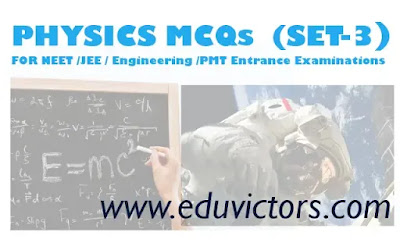## JEE NEET PHYSICS MCQs (Set-2)

FOR NEET - IIT JEE and Other Engineering or premedical Entrance Examinations

Topics Covered: Units & Dimensions, Error in Measurement

Q1: Which of the following sets cannot enter into the list of fundamental quantities in any system of unit

(a) length, mass and velocity
(b) length, time and velocity
(c) mass, time and velocity
(d) length, time and mass

Q2: The base quantity among the following is:

(a) Speed
(b) Weight
(c) Length
(d) Area## PHYSICS MCQs  (SET-1)

FOR NEET - IIT JEE and Other Engineering or premedical Entrance Examinations

Q1: A neutron with velocity v strikes a stationary deuterium atom, its kinetic energy changes by a factor of

(a) 15/16
(b) 1/2
(c) 2/1
(d) 8/9

Q2: AM radio band has carrier frequency from 500 kHz to 1500 kHz. Assuming a fixed inductance in a simple LC circuit, what is  ratio of highest capacitance to lowest capacitance required to cover this frequency range?

(a) 3
(b) 6
(c) 9
(d) 12## Gravitation - Kepler's Laws Of Planetary Motion

Numerical Problems with Solutions

Question 1: The planet Neptune travels around the Sun with a period of 165 years. Show that radius of orbit is approximately 30 times that of the Earth's orbit, considering their orbits circular.

Let T₁ = T(earth) = 1 year
T₂ = T(Neptune) = 165 years# Physics: Important Discoveries

No. Discovery Scientist Year
1. Proton Rutherford 1919
3. Atom John Dalton 1808
4. Atomic Structure Neil Bohr & Rutherford 1913
7. Raman Effect CV. Raman 1928
8. X-Rays Roentgen 1895
9. Photoelectric effect Albert Einstein 1905
10. Periodic table Mendeleev 1888
11. Nuclear Reactor Anrico Fermi 1942
12. Wireless Telegram Marconi 1901
13. Dynamite Alfred Nobel 1867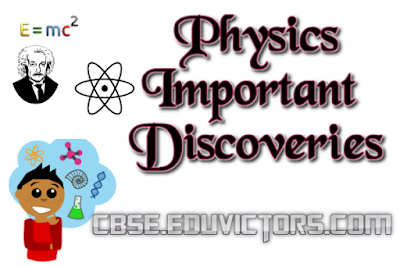## CBSE Class 11 - Physics Sample Question Paper - (2018-19)

Physics is a study of basic laws of nature and their manifestation in different natural phenomena. Physics is the study of physical world and matter and its motion through space and time, along with related concepts such as energy and force. The class 11 syllabus provides logical sequencing of the ‘Units’ of the subject matter with proper placement of concepts with their linkages for better understanding.

Here follows the class 11 Half Year Physics sample question paper to assess your knowledge.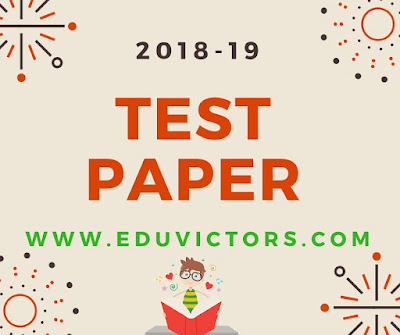## Physics Quiz (1 Word Answer)

Q1: Who firstly demonstrated experimentally the existence of electromagnetic wave?

Q2: The working of the quartz crystal in the watch is based on which effect?

Q3: What is the unit of Radioactivity?

Q4: What is the wavelength of the visible spectrum?

Q5: One astronomical unit is the average distance between___?

Q6: Number of basic S.I. units are ___.

Q7: What is the name of the scientist who stated that matter can be converted into energy?

Q8: The size of the atomic nucleus is of the order of___?

Q9: A temperature at which both the Fahrenheit and the centigrade scales have the same value?## Class 11 - Physics - CHAPTER – 1 PHYSICAL WORLD (Q and A)

Q1: Who gave general theory of relativity?

Answer: Albert Einstein gave the general theory of relativity.

Q2: Is gravitational force is universal?

Answer: Yes, gravitational force is universal.

Q3: How a strong nuclear force is stronger comparing to electromagnetic force?

Answer: A strong nuclear force is 100 times stronger than the electromangnetic force in strength.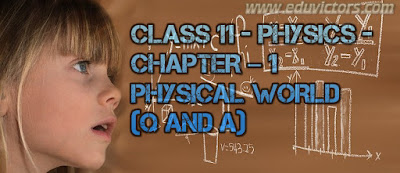## Class XI - PHYSICS - PROJECTILE MOTION - (Assignment)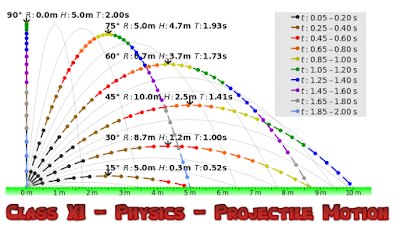image credits: cmglee (Wikimedia)

1. An aeroplane is flying in horizontal direction with a velocity of 600 km/h and at a height of 1960 m. When it is vertically above the point A on the ground, a body is dropped from it. The body strikes the ground at a point B. Calculate distance AB.

2. A body is thrown horizontally from the top of a lower and strike the ground in 3 seconds at an angle 45° with the horizontal. Find the height of the lower and the speed with which it was projected.
[Answer:  44.1 m , 29.4 m/s ]

3. An air craft is flying at a height of 3,500 m above the ground. If the angle subtended at a ground observation point by the air craft positions 10 s apart is 30° while passing over his head, what is the speed of the air craft?## CENTRE OF MASS AND ROTATIONAL MOTION (ASSIGNMENT)

1. Two bodies of masses 0.5 kg and 1 kg are lying in XY plane at (-1,2) and (3,4) respectively. What are the co-ordinates of the centre of mass?

2. Three points masses of 1 kg, 2 kg and 3 kg lie a (1,2), (0,-1) and (2,-3) respectively. Calculate the co-ordinates of the centre of mass of the system.

3. Three identical spheres each of radius r, and mass m are placed touching each other on a horizontal floor. Locate the position of centre of mass of the system.

4. Two particles of mass 2 kg and 1 kg are moving along the same line with speeds 2m/s and 5m/s respectively. What is the speed of the centre of mass of the system. if both the particles are moving
(a) in same direction
(b) in opposite direction?

Answer: 3m/s, 1/3 m/s in the direction of 1 kg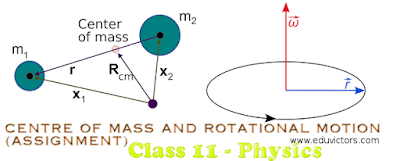## Kepler's Laws

Class 11 - Physics - Gravitation

Kepler's Laws

There has always been a great interest in the motion of planets. Part of the reason is that some people believe that it changed the course of science and laid foundation to Newtonian Laws. By 16th century, a lot of data on the motion of planets had been collected by many astronomers. Based on that data Johannes Kepler, after a life time study, worked out three empirical laws which govern the motion of planets and are known as Kepler's laws of planetary motion.

The laws for planets orbiting the sun, they hold equally well for satellites, either natural or artificial, orbiting Earth or any other massive central body.

The three laws are:

The law of orbits
Law of areas
Law of periods## Understanding Mass and Weight

Physics - Gravitation

Mass and Weight
The quantity of matter in a body is known as mass of the body. Mass is quantitative measure of inertia. Mass is an intrinsic property of matter and does not change as an object is moved from one location to another. Weight, in contrast, is the gravitational force that earth exerts on the object and can vary, depending on how far the object is above the earth's surface or whether it is located near another body such as the moon. The relation between weight W and mass m can be written in one of two ways:

W = GMₑm / r²
W = mg

According to the Newton's second law,
Force = mass ✕ acceleration

or mass = force / acceleration

Mass is of two types :
i) Inertial mass

ii) Gravitational mass

1. Inertial Mass:
Ratio of the applied external force and the acceleration produced in the body is known as inertial mass,

mi = External force applied / Acceleration produced.